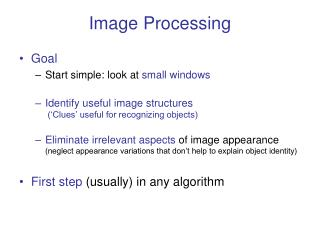DownloadDownload PresentationImage Processing

# Image Processing

Download Presentation## Image Processing

- - - - - - - - - - - - - - - - - - - - - - - - - - - E N D - - - - - - - - - - - - - - - - - - - - - - - - - - -
##### Presentation Transcript

1. Image Processing • Goal • Start simple: look at small windows • Identifyusefulimage structures (‘Clues’ useful for recognizing objects) • Eliminate irrelevant aspects of image appearance (neglect appearance variations that don’t help to explain object identity) • First step (usually) in any algorithm

2. We don’t “see” most information in image

3. We don’t “see” most information in image + Can add invisible change

4. We don’t “see” most information in image + Can add invisible change =

5. Initial Image • Important information small fraction of total

6. Important information Initial Image • Important information small fraction of total Boundary locations boundary sharpness size/direction of brightness change

7. Elder: IJCV 34(2/3), 97–122 (1999) Important information Initial Image • Important information small fraction of total Boundary locations edge sharpness; size/direction of brightness change Reconstruct good semblance of original image just using info at boundaries Reconstruction

8. Image Processing • Goal • Small windows • Identify useful image structures (`clues’) • Eliminate irrelevant aspects • Example 1 • Emphasize signal; suppress noise

9. Image Processing • Goal • Small windows • Identify useful image structures (`clues’) • Eliminate irrelevant aspects • Example 2 • Detect“boundaries” (jumps in brightness) • De-emphasizeslow variations in brightness

10. Why look at small windows? • Local redundancy • Neighboring image points strongly related (points on same object have similar color, texture) Don’t need to know brightness at every pixel  Isolate just useful information (eg, average brightness in window) What’s behind the patch?

11. Why look at small windows? • Details are important! Boundary signalspresence of some object

12. Note • All image locations are equal • Mannequin equally likely to be anywhere in image

13. Note • All image locations are equal* • Mannequin equally likely to be anywhere in image *Not quite true for photographs (people center and compose images)

14. Conclusion • Analyze image over every small window ... ... ...

15. Conclusion • Analyze image over every small window • Easiest: linear weighted sum of brightness in each window ... ... ...

16. Conclusion • Analyze image over every small window • Easiest: linear weighted sum of brightness in each window Convolution (filtering) ... ... ...

17. What is image filtering? For each pixel, modify value based on values of pixels nearby

18. Linear Filtering • New pixel value =weightedsum of nearby pixels • Uses: • Integrate information over regions • Clean up noisy images • Analyze image at different resolutions • Detectimage patterns, brightness boundaries • Connects to Fourier analysis

19. Example (animated) • 1D “image” Average Sum nearby pixels with weights Called mask, kernel, filter… I' Filtered Image Averaging makes filtered curve smoother

20. 2D Linear Filtering 1 1 1 1 1 1 1 1 1 3 x 3filter (averaging or box filter) Image Note: computer vision filters usually have small number of pixels

21. 2D Linear Filtering Filtered Image Image

22. 2D Linear Filtering Filtered Image Image

23. 2D Linear Filtering Filtered Image Image

24. 2D Linear Filtering Filtered Image Image

25. 2D Linear Filtering Filtered Image Image

26. 2D Linear Filtering Filtered Image Image

27. 2D Linear Filtering Filtered Image Image

28. 2D Linear Filtering Filtered Image Image

29. Filtering Equations (correlation!) Filtered image Original image filter (or mask, kernel…)

30. Filtering Equations (correlation!) Filtered image Original image filter is a “little image” containing the weights with which the pixels of are summed • Procedure • For each filter position • Multiply filter and image entries in corresponding positions • Sum and record result at position under filter center

31. Filtering Equations (correlation!) Filtered image Original image filter

32. Filtering Equations (correlation!) Filtered image Original image filter Index ranges give filter size, here (2N+1) x (2N+1)

33. Filtering Equations (correlation!) Filtered image Original image filter Index ranges give filter size, here (2N+1) x (2N+1) Easiest: use odd sized filters, symmetric index range [–N,N] so filter center at (0,0)

34. One Dimension (correlation!) Filtered image For filter of size (2N+1) centered on (0,0)

35. Convolution • Like Correlation withFilter Reversed 1D 2D

36. Convolution ‘-’ instead of ‘+’ crucial change! • Like Correlation withFilter Reversed 1D 2D

37. Convolution • Like Correlation withFilter Reversed • Many nice properties-a kind of multiplication 1D 2D

38. Convolution From now on, linear filtering equals convolution (unless I say otherwise)

39. Convolution procedure Convolved image Original image filter • Procedure • For each filter position • Flip (reflect) filter in both x and y directions • Multiply filter and image entries in corresponding positions • Sum and record result at position under filter center

40. Convolution: symmetric form(doesn’t work for correlation) 1D Convention: extend filter. Assign W(i)=0 for out-of-range

41. Convolution: symmetric form(doesn’t work for correlation) 1D Convention: extend filter. Assign W(i)=0 for out-of-range

42. Convolution: symmetric form(doesn’t work for correlation) 1D This extends for any number of convolutions (again, with convention that everything out of range is zero for W and V)

43. Convolution like multiplication! • Commutative • Associative • Distributive (linear)

44. Convolution like multiplication! • Commutative

45. Convolution like multiplication! • Commutative